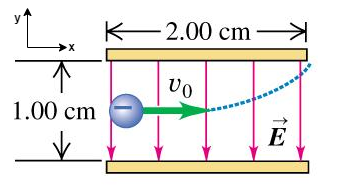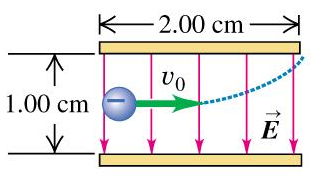# Problem: An electron is projected with an initial speed 1.20×106 m/s into the uniform field between the parallel plates in the figure. Assume that the field between the plates is uniform and directed vertically downward, and that the field outside the plates is zero. The electron enters the field at a point midway between the plates. If the electron just misses the upper plate as it emerges from the field, find the speed of the electron as it emerges from the field?

🤓 Based on our data, we think this question is relevant for Professor Di Credico, Kelley & Smith's class at NEU.

###### FREE Expert Solution

Uniform accelerated motion (UAM) equations:The velocity in the x-direction remains constant. The electron will also acquire a velocity in the y-direction due to the electric field.

We'll determine the time the electron remains in the electric field from the horizontal motion:

Time, t  = Δx/v0

t = (2.00 × 10-2m)/(1.20×106m/s) = 1.67 × 10-8s###### Problem Details

An electron is projected with an initial speed 1.20×106 m/s into the uniform field between the parallel plates in the figure. Assume that the field between the plates is uniform and directed vertically downward, and that the field outside the plates is zero. The electron enters the field at a point midway between the plates. If the electron just misses the upper plate as it emerges from the field, find the speed of the electron as it emerges from the field?